Function Repository Resource:

# CommonMultiples

Find the common multiples of two or more integers

Contributed by: Wolfram|Alpha Math Team
 ResourceFunction["CommonMultiples"][n,m] gives the first m multiples of an number n. ResourceFunction["CommonMultiples"][{n1,n2,…},m] gives the first m common multiples of the numbers ni. ResourceFunction["CommonMultiples"][input] gives the first 10 multiples. ResourceFunction["CommonMultiples"][…,"Grid"] displays the multiples in a grid format.

## Details and Options

ResourceFunction["CommonMultiples"] works not only with integers but also with rational numbers, both real and complex.
The numbers in the first argument of ResourceFunction["CommonMultiples"] must be exact, not approximate, numbers.

## Examples

### Basic Examples (4)

Compute the first ten multiples of an integer:

 In:=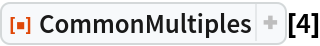Out=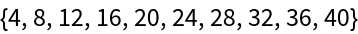Compute the first 10 common multiples of a list of integers:

 In:=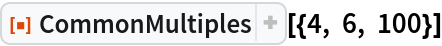Out=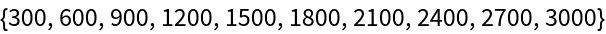Compute only four common multiples:

 In:=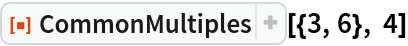Out=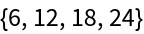Make a grid of common multiples:

 In:=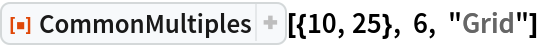Out=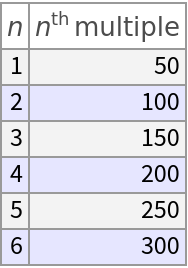### Scope (2)

CommonMultiples can accept fractions:

 In:=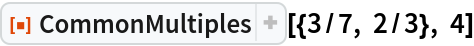Out=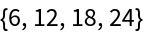CommonMultiples can accept complex numbers:

 In:=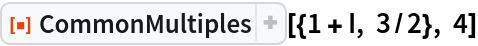Out=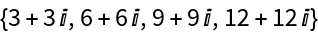### Possible Issues (2)

The numbers in the first argument of CommonMultiples must be exact, not approximate, numbers:

 In:=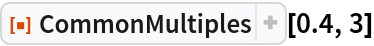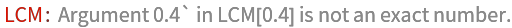Out=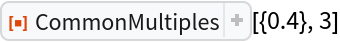CommonMultiples additionally requires its inputs to be rational:

 In:=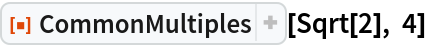Out=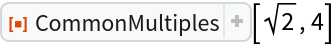In:=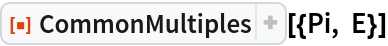Out=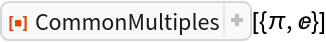## Publisher

Wolfram|Alpha Math Team

## Version History

• 5.0.0 – 23 March 2023
• 4.0.0 – 16 April 2020
• 3.0.0 – 24 January 2020
• 2.0.0 – 06 September 2019
• 1.0.0 – 23 July 2019